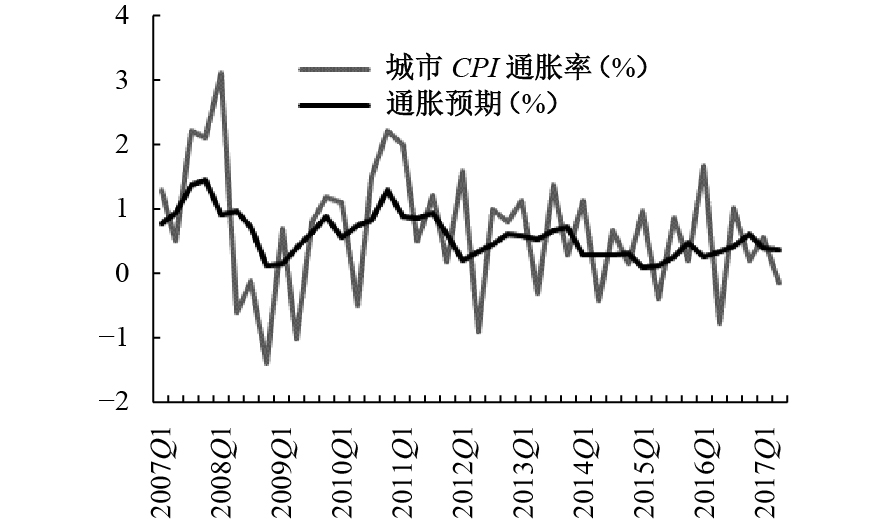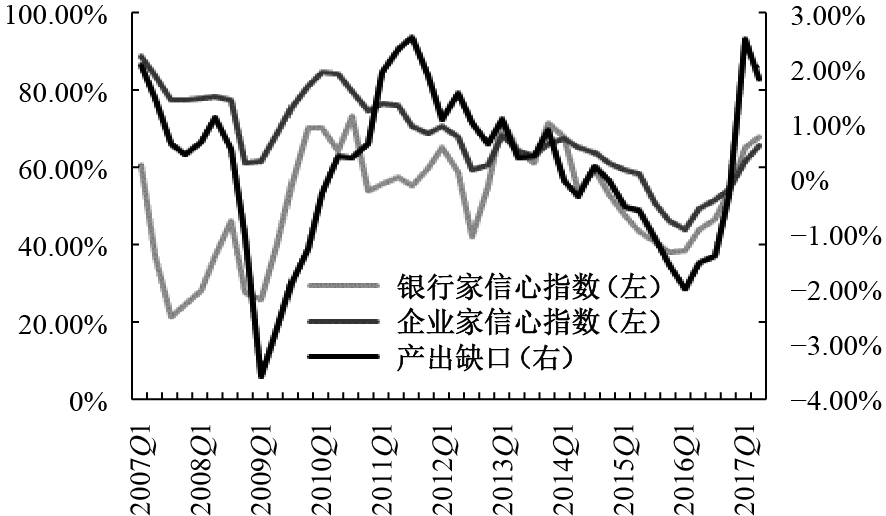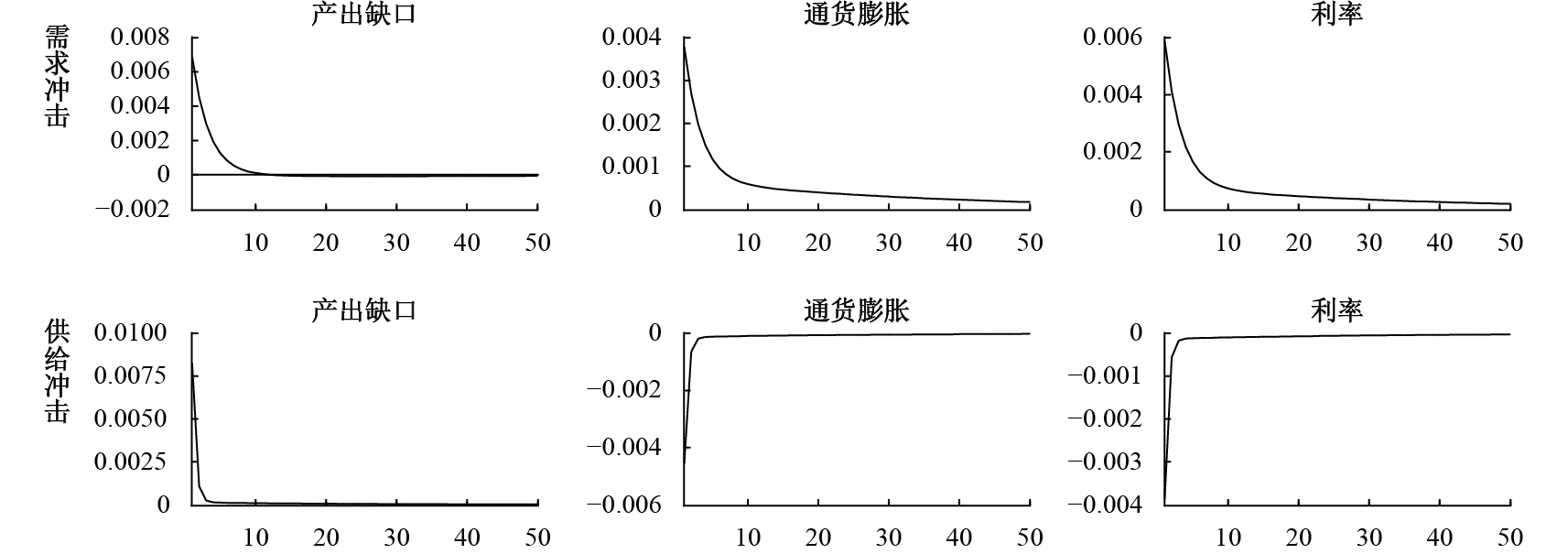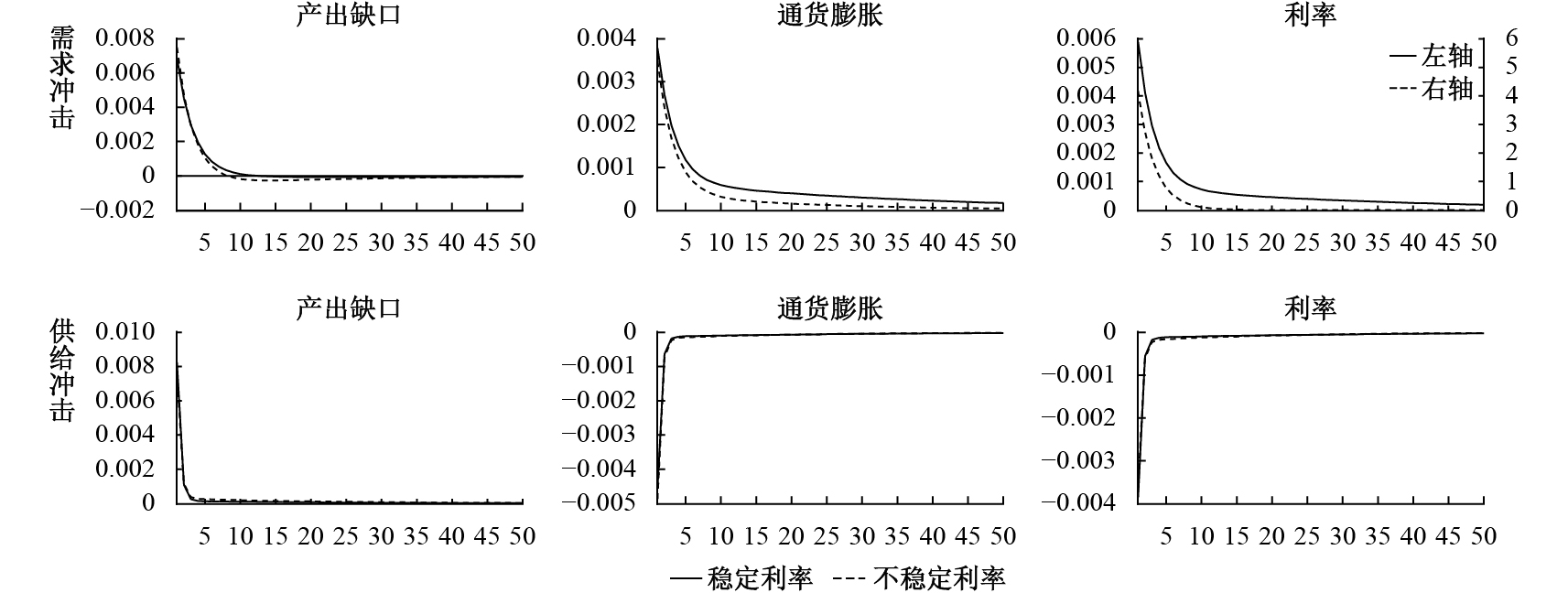﻿ 供求冲击、异质性预期与货币政策范式选择《财经研究》
2019第45卷第3期

1. 上海对外经贸大学 国际经贸学院，上海 201620;
2. 南京大学 经济学院，江苏 南京 210093

Shocks of Supply and Demand，Idiosyncratic Expectations and the Choice of Monetary Policy Paradigms
Gao Jiechao1, Yang Yuanyuan2, Fan Conglai2
2. School of Economics，Nanjing University，Nanjing 210093，China
Key words: idiosyncratic expectations    new Keynesian model    monetary policy

2008年国际金融危机以来，各国宏观调控重拾相机抉择范式。美国迅速调整政策导向，相机实施了多轮量化宽松政策，有力支持了经济复苏。时至今日，学术界对于相机抉择和事前承诺范式孰优孰劣仍莫衷一是，但相机调控的灵活性和针对性较强，这对于经济走出衰退泥潭无疑具有重要作用。2017年中国《政府工作报告》中关于货币政策的表述首次强调要加强相机调控，2018年国务院常务会议再次重申宏观政策要根据形势变化相机预调微调。在经济传导机制梗阻和政策目标多元化背景下，相机调控符合中国当前的国情（陈彦斌，2016）。图 1 城市CPI通胀率与通胀预期图 2 宏观经济信心指数与产出缺口波动

（一）家庭。假定经济中存在连续统的家庭，他们消费、储蓄并向企业提供劳动。家庭的效用函数和预算约束如下：

 ${U_t} = {E_t}\sum\limits_{i = 0}^\infty {{\beta ^i}\left\{ \frac{{C_{t + i}^{1 - \varsigma }}}{{1 - \varsigma }} - \frac{{N_{t + i}^{1 + \gamma }}}{{1 + \gamma }} \right\}}$ (1)
 ${C_t} + {D_t} = \frac{{{W_t}}}{{{P_t}}}{N_t} + \frac{{R_{t - 1}^D{D_{t - 1}}{P_{t - 1}}}}{{{P_t}}} + {\Pi _t}$ (2)

 ${\hat c_t} = {E_t}{\hat c_{t + 1}} - \frac{1}{\varsigma }(\hat R_t^D - {E_t}{\pi _{t + 1}})$ (3)
 ${\hat w_t} - {\hat p_t} = \gamma {\hat n_t} + \varsigma {\hat c_t}$ (4)

 ${\hat y_t} = - \frac{1}{\varsigma }(\hat R_t^D - {E_t}{\pi _{t + 1}}) + {E_t}{\hat y_{t + 1}} + {d_t}$ (5)

（二）企业

1. 最终品企业。假设最终品市场为完全竞争结构，代表性的最终品企业购买中间品 ${Y_{j, t}}$ 来生产最终品： ${Y_t} = {\left( {\int_0^1 {Y_{j, t}^{\frac{{\theta - 1}}{\theta }}dj} } \right)^{\frac{\theta }{{\theta - 1}}}}$ ，其中 $\theta > 1$ 表示各种中间品之间的不变替代弹性，中间品需求函数为 ${Y_{j, t}} = {\left( {\displaystyle\frac{{{P_{j, t}}}}{{P{}_t}}} \right)^{ - \theta }}{Y_t}$ 。由零利润条件可得最终品价格方程： ${P_t} = {\left( {\int_0^1 {P_{j, t}^{1 - \theta }dj} } \right)^{\frac{1}{{1 - \theta }}}}$

2. 中间品企业。假设中间品市场为垄断竞争结构，由区间 $\left( {0, 1} \right)$ 上的连续统企业构成，忽略短期内资本存量的影响，假设代表性企业的生产函数为 ${Y_{j, t}} = {A_t}{N_{j, t}}$ ，其中 ${A_t}$ 为中性技术。厂商通过选择劳动要素来最小化成本函数 $\left( {\displaystyle\frac{{{W_t}}}{{{P_t}}}} \right){N_{j, t}} - {\lambda _{j, t}}({Y_{j, t}} - {A_t}{N_{j, t}})$ ，其中 ${\lambda _{j, t}}$ 为拉格朗日乘子。通过优化求解可得企业的真实边际成本： $M{C_{j, t}} = \displaystyle\frac{{{W_t}}}{{{P_t}{A_t}}}$

 $P_t^* = \frac{\theta }{{\theta - 1}}{E_t}\sum\limits_{i = 0}^\infty {{{\left( {\beta \omega } \right)}^i}M{C_{j, t + i}}}$ (6)

 ${\pi _t} = \beta {E_t}{\pi _{t + 1}} + \delta {\hat y_t} + {s_t}$ (7)

（三）货币当局。与多数DSGE文献直接给定利率规则的表达式不同，本文在经济的总需求约束（动态IS曲线）和总供给约束（NKPC）下，通过优化方法推导货币当局的最优利率规则。传统基于通胀和产出缺口的双因素损失函数假设货币当局只关注实际经济波动，忽视了货币政策调整本身所引致的成本，不符合稳健中性的政策取向，而且基于双因素损失函数推导最优货币政策规则时并不受经济总需求约束（卡尔∙瓦什，2012），这显然有悖于现实。为此，本文借鉴Woodford（2003）的思路，构建包含通胀、产出缺口和利率在内的三因素损失函数，以综合考虑货币当局对经济波动和利率稳定的关注。利率波动也会引起损失，因而利率调整受到限制，此时经济的总需求约束可以对货币当局的最优决策产生实质性影响。三因素损失函数如下：

 $Los{s_t} = f\left( {\pi _t^2, \vartheta \hat y_t^2, \sigma \hat i_t^2} \right)$ (8)

1. 相机抉择。在经济总需求和总供给约束下，货币当局每期都会对损失函数进行最优化，得到当期最优的利率调控规则。此时，最优货币政策决策变为如下的单期优化问题：

 \begin{gathered} \min \begin{aligned} {}&{} \end{aligned}\pi _t^2 + \vartheta \hat y_t^2 + \sigma \hat i_t^2 \hfill \\ s.t.\left\{ {\begin{aligned} & {{{\hat y}_t} = - \displaystyle\frac{1}{\varsigma }({{\hat i}_t} - {E_t}{\pi _{t + 1}}) + {E_t}{{\hat y}_{t + 1}} + {d_t}} \\ & {{\pi _t} = \beta {E_t}{\pi _{t + 1}} + \delta {{\hat y}_t} + {s_t}} \end{aligned}} \right. \hfill \\ \end{gathered} (9)

 $\hat i_t^{} = \frac{{\vartheta + {\delta ^2} + \beta \delta \varsigma }}{{\vartheta + {\delta ^2} + \sigma {\varsigma ^2}}}{E_t}{\pi _{t + 1}} + \frac{{\varsigma \left( {\vartheta + {\delta ^2}} \right)}}{{\vartheta + {\delta ^2} + \sigma {\varsigma ^2}}}{E_t}{\hat y_{t + 1}} + \frac{{\varsigma \left( {\vartheta + {\delta ^2}} \right)}}{{\vartheta + {\delta ^2} + \sigma {\varsigma ^2}}}{d_t} + \frac{{\delta \varsigma }}{{\vartheta + {\delta ^2} + \sigma {\varsigma ^2}}}{s_t}$ (10)

2. 事前承诺。在总需求和总供给约束下，货币当局通过最小化当期和未来损失函数的贴现值，得到最优货币政策。为简便考虑，假定货币当局的预期贴现因子与公众相同。此时，最优货币政策决策变为如下的跨期优化问题：

 \begin{gathered} \min \begin{aligned} {}&{} \end{aligned}{E_t}\sum\limits_{s = 0}^\infty {{\beta ^s}(\pi _{t + s}^2} + \vartheta \hat y_{t + s}^2 + \sigma \hat i_t^2) \hfill \\ s.t.\left\{ {\begin{aligned} & {{{\hat y}_t} = - \frac{1}{\varsigma }({{\hat i}_t} - {E_t}{\pi _{t + 1}}) + {E_t}{{\hat y}_{t + 1}} + {d_t}} \\ & {{\pi _t} = \beta {E_t}{\pi _{t + 1}} + \delta {{\hat y}_t} + {s_t}} \end{aligned}} \right. \hfill \\ \end{gathered} (11)

 {\begin{aligned} \hat i_t^{} = & {\displaystyle\frac{{\varsigma \sigma \left( {\delta + \varsigma } \right)}}{{\beta \left( {\vartheta + {\delta ^2} + \sigma {\varsigma ^2}} \right)}}{{\hat i}_{t - 1}} + \frac{{\vartheta + {\delta ^2} + \beta \delta \varsigma }}{{\vartheta + {\delta ^2} + \sigma {\varsigma ^2}}}{E_t}{\pi _{t + 1}} + \frac{{\varsigma \left( {\vartheta + {\delta ^2}} \right)}}{{\vartheta + {\delta ^2} + \sigma {\varsigma ^2}}}{E_t}{{\hat y}_{t + 1}}} \\ & { + \displaystyle\frac{{\delta \varsigma }}{{\vartheta + {\delta ^2} + \sigma {\varsigma ^2}}}{\pi _{t - 1}} + \frac{{\varsigma \left( {\vartheta + {\delta ^2}} \right)}}{{\vartheta + {\delta ^2} + \sigma {\varsigma ^2}}}{d_t} + \frac{{\delta \varsigma }}{{\vartheta + {\delta ^2} + \sigma {\varsigma ^2}}}{s_t}} \end{aligned}} (12)

（12）式表明，在利率波动进入货币当局的损失函数后，基于事前承诺的利率调整会使最优利率规则内生地表现出平滑机制，而基于双因素损失函数的最优利率规则没有这一特征。与（11）式的相机抉择相比，基于事前承诺的利率调整不仅表现出平滑机制，而且还对上一期通胀做出反应，因此历史通胀会影响当期利率调整。

（四）预期机制。为避免参数过多而导致参数估计不可识别以及参数校准过于主观，本文参照Gelain等（2013）、许志伟等（2015）等在模型中引入理性预期和简单适应性预期加权设定的方式，刻画更加贴近现实的异质性预期，并以理性预期情形为基准，模拟异质性预期下货币政策调控效果的变化情况。

1. 理性预期。理性预期假说认为公众具有高度理性，能够通过自我学习和调整，最终实现对经济变量的无偏和一致估计，公众能够准确预测随机冲击以外的经济变量的基本走势。为简便考虑，与多数DSGE文献的做法一致，采用如下完美信息条件下的理性预期假定：

 $E_t^R{X_{t + 1}} = {X_{t + 1}}$ (13)

2. 异质性预期。本文构建的异质性预期同时包含了理性预期机制和适应性预期机制。借鉴Gelain等（2013）的预期设置方式，在适应性预期下，公众对未来的预期由过去形成的预期以及过去预期与实际值偏差的加成得到：

 $E_t^A{X_{t + 1}} = E_{t - 1}^A{X_t} + \lambda \left( {{X_t} - E_{t - 1}^A{X_t}} \right)$ (14)

 $E_t^H{X_{t + 1}} = \kappa E_t^A{X_{t + 1}} + \left( {1 - \kappa } \right)E_t^R{X_{t + 1}}$ (15)

（一）参数校准。在贝叶斯估计之前，必须对模型中的部分参数进行校准，否则无法识别模型中的全部参数。在参数校准过程中，同时确保模型存在唯一稳定均衡解。与多数文献一样，将家庭主观贴现因子 $\beta$ 的季度值校准为0.99。参照马勇和陈雨露（2014）的估计结果，取居民消费跨期替代弹性的倒数 $\varsigma = 0.451$ 。研究中国问题的已有文献对劳动供给弹性的倒数 $\gamma$ 取值差异较大，王国静和田国强（2014）注意到这一问题，以他们的估计结果将 $\gamma$ 设定为2.2329。借鉴郭豫媚等（2016）的研究，取价格调整的粘滞程度参数 $\omega = 0.75$

（二）数据选择。由于模型系统包含需求冲击和供给冲击2个外生冲击，本文选择产出（以实际GDP衡量）和通货膨胀（以CPI变化率衡量）这两个观测变量，数据来自中经网统计数据库，时间区间为2000年第1季度至2017年第1季度。其中，真实产出由CPI平减得到，变量采用X-12方法进行季节调整，利用一阶差分去除趋势。此外，使用金融机构1年期法定存款基准利率的季度数据表示无风险存款利率。

（三）先验分布设置。适应性预期中纠错参数 $\lambda$ 的设定与许志伟等（2015）类似，令其服从均值为0.3、标准差为0.2的Beta分布。异质性预期中的适应性预期成分参数 $\kappa$ 介于0和1之间，设其服从均值为0.5、标准差为0.2的Beta分布。将货币当局损失函数中的产出缺口权重参数 $\vartheta$ 设为0.5，令其服从标准差为0.2的Normal分布。参照Woodford（2003）的研究，设定货币当局损失函数中的利率权重参数 $\sigma$ 服从均值为0.236、标准差为0.2的Normal分布。参考王国静和田国强（2014）、马勇和陈雨露（2014）等文献，将需求和供给冲击的持久性参数 ${\rho _d}$ ${\rho _s}$ 分别设为服从均值为0.5、标准差为0.2的Beta分布，将标准差 ${\sigma _d}$ ${\sigma _s}$ 分别设为服从均值为0.01的Inv_Gamma分布。参数的先验分布及贝叶斯估计结果见表1

 参数 先验分布 Model1 Model2 Model3 Model4 后验 均值 95% 置信区间 后验 均值 95% 置信区间 后验 均值 95% 置信区间 后验 均值 95% 置信区间 ${\kappa }$ Beta[0.5，0.2] 0.3209 [0.0481，0.6134] 0.4440 [0.1940，0.6489] ${\lambda }$ Beta[0.3，0.2] 0.2137 [0.0004，0.6200] 0.0786 [0.0001，0.1635] ${\vartheta }$ Normal[0.5，0.2] 0.0527 [0.0126，0.0886] 0.5084 [0.2770，0.7463] 0.0563 [0.0074，0.1010] 0.5817 [0.3197，0.8530] ${\sigma }$ Normal[0.236，0.2] 0.3611 [0.2662，0.4803] 0.4474 [0.2079，0.6697] 0.4034 [0.3189，0.4914] 0.4544 [0.2150，0.7050] ${{\rho _d}}$ Beta[0.5，0.2] 0.6414 [0.5389，0.7520] 0.7925 [0.6944，0.8956] 0.5541 [0.3794，0.7610] 0.7964 [0.6957，0.9067] ${{\rho _s}}$ Beta[0.5，0.2] 0.1668 [0.0277，0.2832] 0.5533 [0.4267，0.7032] 0.1450 [0.0178，0.2487] 0.2533 [0.0775，0.4169] ${{\sigma _d}}$ Inv_Gamma[0.01，∞) 0.0149 [0.0078，0.0211] 0.0715 [0.0339，0.1128] 0.0146 [0.0075，0.0227] 0.0702 [0.0321，0.1126] ${{\sigma _s}}$ Inv_Gamma[0.01，∞) 0.0059 [0.0048，0.0070] 0.0045 [0.0031，0.0059] 0.0058 [0.0042，0.0070] 0.0062 [0.0051，0.0074] 注：Model1为理性预期下的相机抉择模型，Model2为理性预期下的事前承诺模型，Model3为异质性预期下的相机抉择模型，Model4为异质性预期下的事前承诺模型。

（一）模型评价。首先，对模型与实际经济的拟合程度进行评价，甄别何种货币政策范式和预期形成机制对现实经济的解释力度更强。表2从标准差、一阶自相关系数以及与产出缺口的相关系数三个维度报告了模型与实际经济的拟合程度。整体来看，对于与产出缺口的相关系数，Model2和Model4的表现与实际经济特征出入很大，表明事前承诺范式不符合中国经济运行现实；对于一阶自相关系数和标准差，Model1和Model3的拟合效果较为接近，表明中国经济运行呈现出相机抉择特征。进一步从表1的贝叶斯估计结果来看，Model3估计出的异质性预期中的适应性预期成分参数 $\kappa$ 和适应性预期中的纠错参数 $\lambda$ 在95%的置信区间内显著不为零，分别达到32%和21%。这与许志伟等（2015）估计出中国公众预期中的适应性成分约为80%的结论比较接近，进一步表明中国的预期机制的确存在较强的适应性特征。因此，中国的货币政策表现出明显的相机抉择倾向，宏观经济预期呈现出理性预期和适应性预期混合的异质性特征。

 标准差 实际经济 Model1 Model2 Model3 Model4 产出缺口 0.0119 0.0121 0.0119 0.0124 0.0116 通货膨胀 0.0071 0.0071 0.0095 0.0076 0.0078 利率 0.1132 0.0077 0.0359 0.0970 0.0379 一阶自相关系数 实际经济 Model1 Model2 Model3 Model4 产出缺口 0.2867 0.3868 0.2570 0.4197 0.2577 通货膨胀 0.3906 0.4601 0.6385 0.4442 0.3624 利率 0.1507 0.5196 0.8516 0.6493 0.8604 与产出缺口的相关系数 实际经济 Model1 Model2 Model3 Model4 产出缺口 1 1 1 1 1 通货膨胀 0.0646 0.0750 −0.1694 0.1113 0.1192 利率 0.1953 0.1957 −0.0150 0.3549 −0.2201图 3 异质性预期下相机抉择模型动态

 需求冲击 供给冲击 产出缺口 54.54% 45.46% 通货膨胀 62.89% 37.11% 利率 83.63% 16.37% 产出缺口预期 55.56% 44.44% 通货膨胀预期 66.18% 33.82%

（二）预期和范式转变对货币政策稳定经济效果的影响。我们将分析当预期由异质性预期转变为理性预期，或者相机抉择范式转变为事前承诺范式时，货币政策的经济稳定效果会如何变化？模拟结果见图4图 4 不同预期和范式组合下的模型动态

1. 需求冲击。从产出缺口的动态响应路径来看，异质性预期下的相机抉择模型向稳态收敛的速度最快，这符合近年来我国始终将“稳增长”作为宏观调控的重中之重的基本事实；而无论是在理性预期还是异质性预期下，事前承诺下的波动幅度都偏高，收敛速度也更慢。从通货膨胀的动态响应路径来看，理性预期下的相机抉择模型收敛速度最快，异质性预期下的粘滞程度大、难以迅速向稳态回归，尤其是异质性预期下的相机抉择模型。利用2000年第1季度至2017年第1季度的实际数据分析发现，通货膨胀对稳态的偏离明显高于产出缺口，再次印证了当前我国异质性预期下的相机抉择调控具有“重产出、轻通胀”的特征。从利率的动态响应路径来看，相机抉择下的收敛速度最快，其中理性预期下的粘滞程度又低于异质性预期，而事前承诺下的波动幅度大、粘滞程度高、难以迅速向稳态回归，其中理性预期下的情况稍优于异质性预期。

2. 供给冲击。从产出缺口的动态响应路径来看，与事前承诺相比，相机抉择下的粘滞程度更低、回归稳态的速度更快，而理性预期下的事前承诺模型的粘滞程度最高、收敛速度最慢。从通货膨胀的动态响应路径来看，相机抉择下的波动幅度最小、收敛速度最快，而理性预期下的事前承诺模型粘滞性最强。从利率的动态响应路径来看，理性预期下的相机抉择模型收敛速度最快，其次是异质性预期下的相机抉择，而事前承诺虽然在异质性预期下的波动幅度小于理性预期，但其粘滞性更强、难以迅速回归稳态。这与需求冲击情形下的结果是基本吻合的，表明“理性预期+相机抉择”组合的利率稳定效果最佳，而“异质性预期+事前承诺”组合的利率稳定效果最差。这再次说明在公众预期没有明显改善的条件下，货币政策不宜盲目转向事前承诺范式。

（三）稳定利率操作的福利效应。本文的贝叶斯估计结果显示，货币当局损失函数中的利率权重参数 $\sigma$ 为0.4034，表明中国的货币政策实施确实考虑了利率稳定因素，反映了货币政策的中性基调及其对金融稳定的关注。为分析是否纳入稳定利率操作对货币政策调控效果的影响，取 $\sigma$ =0.001，表示货币政策不采取稳定利率操作，并与 $\sigma$ =0.4034的基准情形进行比较分析。图5可知，在需求冲击下，不采取稳定利率操作会导致利率波动大幅增加，而对产出缺口和通货膨胀波动的抑制效果更好；在供给冲击下，是否采取稳定利率操作的经济波动效应差别很小。图 5 稳定利率操作的经济波动效应

 是否采取稳定利率操作 需求冲击 供给冲击 否（ $\sigma$ =0.001） 0.0302 3.39937E-05 是（ $\sigma$ =0.4034） 6.9486E-05 3.12648E-05

① 尽管中国处于经济转型期，存在大量的非预期冲击，但是不可否认，经济主体预期的理性程度还不够高。许多研究显示我国是有限理性预期，适应性成分仍比较明显（张蓓，2009；范从来和高洁超，2016）。

② 本文的异质性预期是指代表性主体的预期中同时包含理性成分和适应性成分，而非不同类型行为主体的预期。

③ 当前，迫于不断升级的中美贸易摩擦，中国货币政策有重回稳健的态势，但毋庸置疑，稳健中性才是货币政策的长期取向。2018年10月，中国人民银行网站在沟通交流栏目中重申了稳健中性的货币政策取向保持不变。

④ 根据匿名审稿人的意见，采用HP滤波处理数据会存在DSGE模型变量与原始数据不匹配的问题，本文采用一阶差分滤去变量中的时间趋势；此外，还使用GDP平减指数代替CPI进行贝叶斯稳健性估计，所得结果与原文保持了较高的一致性。

⑤ 由于 $\sigma$ 取0时经济总需求方程无法对货币政策制定形成实际约束，为比较需求冲击下货币政策是否采取稳定利率操作带来的经济波动效应变化，取 $\sigma$ =0.001，近似表示不采取稳定利率操作。

  卞志村, 高洁超. 适应性学习、宏观经济预期与中国最优货币政策[J]. 经济研究, 2014(4): 32–46. DOI:10.3969/j.issn.2095-0977.2014.04.009  卞志村, 高洁超. 宏观稳定视角的货币政策体制设计研究[J]. 金融经济学研究, 2015(2): 3–14.  卞志村, 张义. 央行信息披露、实际干预与通胀预期管理[J]. 经济研究, 2012(12): 15–28. DOI:10.3969/j.issn.1004-7778.2012.12.005  陈彦斌. " 十三五”规划纲要关于宏观调控的新思路[N]. 光明日报, 2016-05-04.  程均丽. 异质预期下的货币政策: 相机还是承诺[J]. 国际金融研究, 2010(3): 18–26.  范从来, 高洁超. 适应性学习与中国通货膨胀非均衡分析[J]. 经济研究, 2016(9): 17–28. DOI:10.3969/j.issn.1672-5719.2016.09.024  郭豫媚, 陈伟泽, 陈彦斌. 中国货币政策有效性下降与预期管理研究[J]. 经济研究, 2016(1): 28–41. DOI:10.3969/j.issn.1002-5863.2016.01.010  郭豫媚, 周璇. 央行沟通、适应性学习和货币政策有效性[J]. 经济研究, 2018(4): 77–91. DOI:10.3969/j.issn.1672-5719.2018.04.070  [美]卡尔·瓦什. 货币理论与政策[M]. 彭兴韵, 曾刚, 译. 上海: 格致出版社, 上海三联书店, 上海人民出版社, 2012.  刘凤良, 鲁旭, 易信. 中国部门间通货膨胀的" 均值回复”特征研究——新方法的构建及实证分析[J]. 管理世界, 2012(9): 36–48.  马勇, 陈雨露. 经济开放度与货币政策有效性: 微观基础与实证分析[J]. 经济研究, 2014(3): 35–46. DOI:10.3969/j.issn.1009-0657.2014.03.008  王国静, 田国强. 金融冲击和中国经济波动[J]. 经济研究, 2014(3): 20–34. DOI:10.3969/j.issn.2095-0977.2014.03.006  肖争艳, 陈彦斌. 中国通货膨胀预期研究: 调查数据方法[J]. 金融研究, 2004(11): 1–18. DOI:10.3969/j.issn.1003-1812.2004.11.001  许志伟, 樊海潮, 薛鹤翔. 公众预期、货币供给与通货膨胀动态——新凯恩斯框架下的异质性预期及其影响[J]. 经济学（季刊）, 2015(4): 1211–1234.  杨源源, 张晓林, 于津平. 异质性预期、宏观经济波动与货币政策有效性——来自数量型和价格型工具的双重检验[J]. 国际金融研究, 2017(9): 25–34.  张蓓. 我国居民通货膨胀预期的性质及对通货膨胀的影响[J]. 金融研究, 2009(9): 40–54. DOI:10.3969/j.issn.1674-5477.2009.09.009  Blanchard O J, Fischer S. Lectures on macroeconomics[M]. Cambridge MA: The MIT Press, 1989.  Branch W A. The theory of rationally heterogeneous expectations: Evidence from survey data on inflation expectations[J]. The Economic Journal, 2004, 114(497): 592–621. DOI:10.1111/j.1468-0297.2004.00233.x  Branch W A, Evans G W. Monetary policy and heterogeneous expectations[J]. Economic Theory, 2011, 47(2−3): 365–393. DOI:10.1007/s00199-010-0539-9  Calvo G A. Staggered prices in a utility-maximizing framework[J]. Journal of Monetary Economics, 1983, 12(3): 383–398. DOI:10.1016/0304-3932(83)90060-0  Cowan K, Filardo A, García P, et al. It in financially stable economies: Has it been flexible enough?[R]. Documentos de Trabajo, 2009.  Evans G W, Honkapohja S. Monetary policy, expectations and commitment[J]. The Scandinavian Journal of Economics, 2006, 108(1): 15–38. DOI:10.1111/sjoe.2006.108.issue-1  Gelain P, Lansing K J, Mendicino C. House prices, credit growth, and excess volatility: Implications for monetary and macroprudential policy[R]. Norges Bank Working Paper, 2013.  Hommes C H, Massaro D, Weber M. Monetary policy under behavioral expectations: Theory and experiment[R]. Bank of Lithuania Working Paper, 2015.  Huang K X D, Liu Z, Zha T. Learning, adaptive expectations and technology shocks[J]. The Economic Journal, 2009, 119(536): 377–405. DOI:10.1111/j.1468-0297.2008.02238.x  Lucas Jr R E. Expectations and the neutrality of money[J]. Journal of Economic Theory, 1972, 4(2): 103–124. DOI:10.1016/0022-0531(72)90142-1  McCallum B T, Nelson E. Timeless perspectives vs. discretionary monetary policy in forward-looking models[R]. NBER Working Paper No.7915, 2000.  Muth J F. Rational expectations and the theory of price movements[J]. Econometrica, 1961, 29(3): 315–335. DOI:10.2307/1909635  Orphanides A, Williams J C. The decline of activist stabilization policy: Natural rate misperceptions, learning, and expectations[J]. Journal of Economic Dynamics and Control, 2005, 29(11): 1927–1950. DOI:10.1016/j.jedc.2005.06.004  Pfajfar D, Zakelj B. Inflation expectations and monetary policy design: Evidence from the laboratory[R]. CentER Discussion Paper Series No.2011-091, 2011.  Sargent T J, Wallace N. Rational expectations and the theory of economic policy[J]. Journal of Monetary Economics, 1976, 2(2): 169–183. DOI:10.1016/0304-3932(76)90032-5  Sargent T J. Bounded rationality in macroeconomics: The Arne Ryde memorial lectures[M]. Clarendon Press, 1993.  Svensson L E O. Inflation targeting as a monetary policy rule[J]. Journal of Monetary Economics, 1999, 43(3): 607–654. DOI:10.1016/S0304-3932(99)00007-0  Taylor J B. The role of policy in the great recession and the weak recovery[J]. American Economic Review, 2014, 104(5): 61–66. DOI:10.1257/aer.104.5.61  Woodford M. Optimal interest-rate smoothing[J]. The Review of Economic Studies, 2003, 70(4): 861–886. DOI:10.1111/1467-937X.00270  Woodford M. Inflation targeting and financial stability[R]. NBER Working Paper No.17967, 2012.